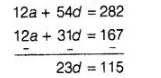# The sum of the first five terms of an AP

Question:

The sum of the first five terms of an AP and the sum of the first seven terms of the same AP is 167. If the sum of the first ten terms of this AP is 235, find

the sum of its first twenty terms.

Solution:

Let the first term, common difference and the number of terms of an AP are a, d and n, respectively.

$\because$ Sum of first $n$ terms of an AP, $S_{n}=\frac{n}{2}[2 a+(n-1) d]$ $\ldots$ (i)

$\therefore$ Sum of first five terms of an AP, $S_{5}=\frac{5}{2}[2 a+(5-1) d]$ [from Eq. (i)]

$=\frac{5}{2}(2 a+4 d)=5(a+2 d)$

$\Rightarrow \quad S_{5}=5 a+10 d$ ....(ii)

and sum of first seven terms of an AP, $S_{7}=\frac{7}{2}[2 a+(7-1) d]$

$=\frac{7}{2}[2 a+6 d]=7(a+3 d)$

$\Rightarrow$ $S_{7}=7 a+21 d$ ....(iii)

Now, by given condition,

$S_{5}+S_{7}=167$

$\Rightarrow \quad 5 a+10 d+7 a+21 d=167$

$\Rightarrow \quad 12 a+31 d=167$ ....(iv)

Given that, sum of first ten terms of this AP is 235 .

$\therefore \quad S_{10}=235$

$\Rightarrow \quad \frac{10}{2}[2 a+(10-1) d]=235$

$\Rightarrow \quad 5(2 a+9 d)=235$

$\Rightarrow \quad 2 a+9 d=47$ .....(v)

On multiplying Eq. (v) by 6 and then subtracting it into Eq. (vi), we get$\Rightarrow \quad d=5$

Now, put the value of $d$ in Eq. (v), we get

$2 a+9(5)=47 \Rightarrow 2 a+45=47$

$\Rightarrow$ $2 a=47-45=2 \Rightarrow a=1$

Sum of first twenty terms of this AP, $S_{20}=\frac{20}{2}[2 a+(20-1) d]$

$=10[2 \times(1)+19 \times(5)]=10(2+95)$

$=10 \times 97=970$

Hence, the required sum of its first twenty terms is 970 .